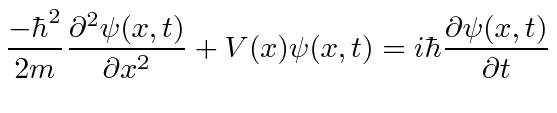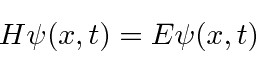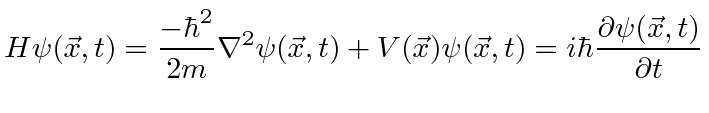## Deriving the Equation from Operators

For a free particle, we haveLets try this equation on our states of definite momentum.The constant in front of the wave function can be removed from both sides. Its there for normalization, not part of the solution. We will go ahead and do the differentiation.Our wave function will be a solution of the free particle Schrödinger equation provided. This is exactly what we wanted. So we have constructed an equation that has the expected wave-functions as solutions. It is a wave equation based on the total energy.

Adding in potential energy, we have the Schrödinger Equationorwhereis the Hamiltonian operator.

In three dimensions, this becomes.We will use it to solve many problems in this course.

So the Schrödinger Equation is, in some sense, simply the statement (in operators) that the kinetic energy plus the potential energy equals the total energy.

Jim Branson 2013-04-22